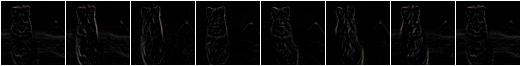# augmenters.convolutional¶

## Convolve¶

Apply a Convolution to input images.

API link: `Convolve`

Example. Convolve each image with a 3x3 kernel:

```import imgaug.augmenters as iaa
matrix = np.array([[0, -1, 0],
[-1, 4, -1],
[0, -1, 0]])
aug = iaa.Convolve(matrix=matrix)
```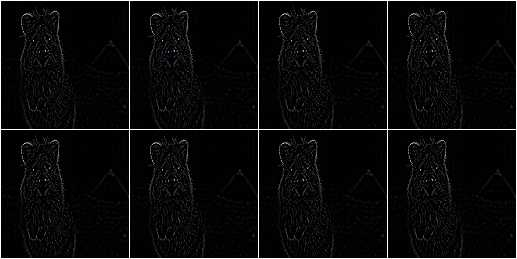Example. Convolve each image with a 3x3 kernel, which is chosen dynamically per image:

```def gen_matrix(image, nb_channels, random_state):
matrix_A = np.array([[0, -1, 0],
[-1, 4, -1],
[0, -1, 0]])
matrix_B = np.array([[0, 0, 0],
[0, -4, 1],
[0, 2, 1]])
if random_state.rand() < 0.5:
return [matrix_A] * nb_channels
else:
return [matrix_B] * nb_channels
aug = iaa.Convolve(matrix=gen_matrix)
```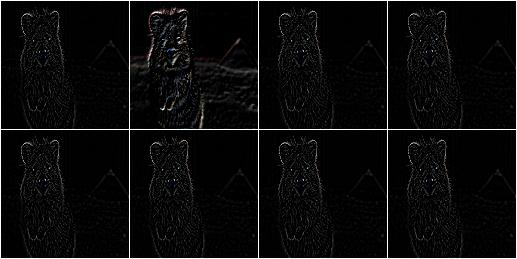## Sharpen¶

Augmenter that sharpens images and overlays the result with the original image.

API link: `Sharpen()`

Example. Sharpen an image, then overlay the results with the original using an alpha between 0.0 and 1.0:

```import imgaug.augmenters as iaa
aug = iaa.Sharpen(alpha=(0.0, 1.0), lightness=(0.75, 2.0))
```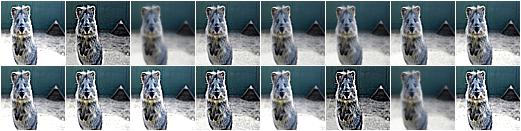Example. Effects of keeping `lightness` fixed at `1.0` and then varying `alpha` between `0.0` and `1.0` in eight steps: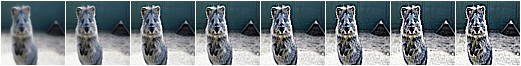Example. Effects of keeping `alpha` fixed at `1.0` and then varying `lightness` between `0.75` and `1.5` in eight steps: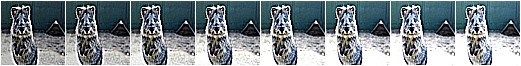## Emboss¶

Augmenter that embosses images and overlays the result with the original image.

API link: `Emboss()`

Example. Emboss an image, then overlay the results with the original using an alpha between `0.0` and `1.0`:

```import imgaug.augmenters as iaa
aug = iaa.Emboss(alpha=(0.0, 1.0), strength=(0.5, 1.5))
```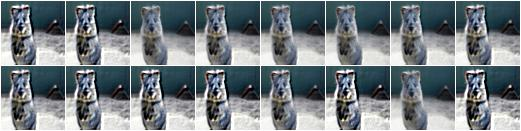Example. Effects of keeping `strength` fixed at `1.0` and then varying `alpha` between `0.0` and `1.0` in eight steps: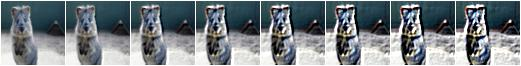Example. Effects of keeping `alpha` fixed at `1.0` and then varying `strength` between `0.5` and `1.5` in eight steps: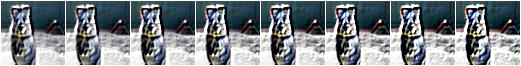## EdgeDetect¶

Augmenter that detects all edges in images, marks them in a black and white image and then overlays the result with the original image.

API link: `EdgeDetect()`

Example. Detect edges in images, turning them into black and white images and then overlay these with the original images using random alphas between 0.0 and 1.0:

```import imgaug.augmenters as iaa
aug = iaa.EdgeDetect(alpha=(0.0, 1.0))
```

Example. Effect of increasing `alpha` from `0.0` to `1.0` in eight steps: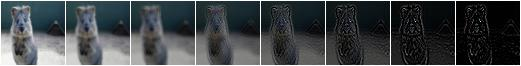## DirectedEdgeDetect¶

Augmenter that detects edges that have certain directions and marks them in a black and white image and then overlays the result with the original image.

API link: `DirectedEdgeDetect()`

Example. Detect edges having random directions (0 to 360 degrees) in images, turning the images into black and white versions and then overlay these with the original images using random alphas between 0.0 and 1.0:

```import imgaug.augmenters as iaa
aug = iaa.DirectedEdgeDetect(alpha=(0.0, 1.0), direction=(0.0, 1.0))
```

Example. Effect of fixing `direction` to 0.0 and then increasing `alpha` from `0.0` to `1.0` in eight steps: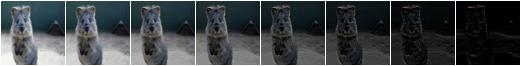Example. Effect of fixing `alpha` to `1.0` and then increasing `direction` from `0.0` to `1.0` (0 to 360 degrees) in eight steps: